# AverageFile: general_blocks/Statistal/Average.sim
Description
The model is used to average a series of real-valued numbers. Each test input will
cause the mean value of all numbers placed on the in port since the most recent clr
signal to be placed on the out port.
Ports
Input Ports
• in       Data Type: REAL
• clr      Data Type: TRIGGER
• test     Data Type: TRIGGER
Output Ports
• avg     Data Type: REAL
Parameters
• none

# Batch_RmaxFile: general_blocks/Statistical/Batch_Rmax.sim
Description
The model extracts the largest real value from a series of real input values.
When the test input is triggered, the largest value since the previous test
input is placed on the maximum output port, and the current maximum
value is reset to a very small value.
Ports
Input Ports
• input     Data Type: REAL
• test      Data Type: TRIGGER
Output Ports
• maximum     Data Type: REAL
Parameters
• none

# Batch_MeanFile: general_blocks/Statistical/Batch_Mean.sim
Description
The model takes a series of REAL value inputs and averages all values which
come in over a batch period. At the end of the batch period, the average will be
written to the output and then the average will be cleared. Inputs are grouped
according to time batches. The duration of each batch is determined by two parameters:
NBatches and StartTime.
Ports
Input Ports
• in     Data Type: REAL
Output Ports
• avg     Data Type: REAL
Parameters
• NBatches     Data Type: INTEGER
• StartTime     Data Type: REAL

# Batch_RminFile: general_blocks/Statistical/Batch_Rmin.sim
Description
The model extracts the smallest real value from a series of real input values.
When the test input is triggered, the smallest value since the previous test
input is placed on the minimum output port, and the current minimum
value is reset to a very large value.
Ports
Input Ports
• input    Data Type: REAL
• test      Data Type: TRIGGER
Output Ports
• minimum     Data Type: REAL
Parameters
• none

# Batch_StatisticsFile: general_blocks/Statistical/Batch_Statistics.sim
Description
The model will output the basic statistics computed from input numbers
separated into different (time) batches. The batches are fixed length in time
and are of equal duration. All inputs which occur before the simulation clock
reaches the value of the StartTime parameter are discarded. After the start time,
the remaining simulation time is divided into equal length batches and the basic
statistics for all values which come in during a batch period are placed on the stats
output port at the end of each batch period.

The simulation time is divided into equal time intervals according to two parameters:
NBatches and StartTime.
Ports
Input Ports
• input    Data Type: REAL
Output Ports
• stats    Data Type: Complex Structure "Basic_statistic"
Parameters
• NBatches              Data Type: INTEGER
• StartTime = 0.0       Data Type: REAL
• NoSampleMean = 0.0
• NoSampleVariance = -1.0

# Batch_TimingFile: general_blocks/Statistical/Batch_Timing.sim
Description
The model generates trigger signals to be used for batch statistic compilation.
The Startup port is enabled when the simulation clock equals the value of the
StartTime parameter. Startup enabled when Tnow = StartTime and Batch enabled
when Tnow = (StartTime + (i * Batch Period)) where i is any integer between 1 and NBatches
Batch Period = (TSTOP - StartTime)/NBatches
Ports
Input Ports
• none
Output Ports
• Startup<    Data Type: TRIGGER
• Batch<      Data Type: TRIGGER
Parameters
• NBatches     Data Type: INTEGER
• StartTime     Data Type: REAL

# Construct_Dimensioned_Stats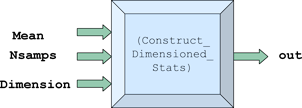File: general_blocks/Statistical/Construct_Dimensioned_Stats.sim
Description
The model creates a Dimensioned Stats data structure and inserts the Mean,
Nsamps, and Dimension fields from the values of the inputs.
Ports
Input Ports
• Mean             Data Type: REAL
• Nsamps          Data Type: INTEGER
• Dimension     Data Type: INTEGER
Output Ports
• out           Data Type: Dimensioned Stats
Parameters
• none

# Construct_TimeAverage_Stats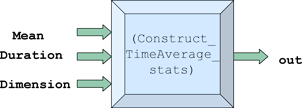File: general_blocks/Statistical/Construct_TimeAverage_Stats.sim
Description
The model creates a time average Statistics data structure and inserts the Mean,
Duration, and Dimension fields from the values of the inputs.
Ports
Input Port
• Mean            Data Type: REAL
• Duration      Data Type: REAL
• Dimension   Data Type: INTEGER
Output Ports
• out     Data Type: time average stats
Parameters
• none

# Dimensioned_Ensemble_Average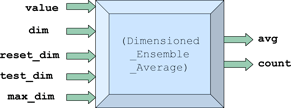File: general_blocks/Statistical/Dimensioned_Ensemble_Average.sim
Description
The model computes an ensemble average for each of several dimensions.
An ensemble average is the sum of all the value inputs for the dimension
divided by the number of inputs in the sum. The number of dimensions must be
set with Max_Dimensions parameter and may be changed by enabling max_dim input.

To access the time average, enable the test_dim input with the desired integer dimension.
For that dimension, the average will be computed and placed on the avg output and the
number of samples in the average is placed on the count output.

To reset dimensions enable the reset_dim input with the desired dimension and all previous
history for the dimension will be cleared.
Ports
Input Ports
• value          Data Type: REAL
• dim             Data Type: INTEGER
• reset_dim   Data Type: INTEGER
• test_dim     Data Type: INTEGER
• max_dim    Data Type: INTEGER
Output Ports
• avg      Data Type: REAL
• count   Data Type: INTEGER
Parameters
• Max_Dimensions = 10   Data Type: INTEGER

# Dimensioned_Time_Average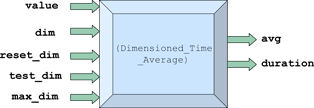File: general_blocks/Statistical/Dimensioneded_Time_Average.sim
Description
The model computes an time average for each of several dimensions.
A time average is the area under a plot of a value versus time divided by the
length of time of the observation.

To access the time average, enable the test_dim input with the desired integer
dimension. For that dimension, the time average will be computed and placed
on the avg output and the duration of time which the Time Average has been
calculated is placed on the duration output.

To reset the time average enable the reset_dim input with the desired dimension
and all previous history for the dimension will be cleared.
Ports
Input Ports
• value          Data Type: REAL
• dim            Data Type: INTEGER
• reset_dim  Data Type: INTEGER
• test_dim    Data Type: INTEGER
• max_dim   Data Type: INTEGER
Output Ports
• avg           Data Type: REAL
• duration   Data Type: REAL
Parameters
• Max_Dimensions = 10   Data Type: INTEGER

# Find_BinFile: general_blocks/Statistical/Find_Bin_Number.sim
Description
The model takes the value which is to be added to the histogram
and computes the bin number into which the value should be placed.
The model outputs the bin number.
Ports
Input Ports
• in   Data Type: REAL
Output Ports
• bin   Data Type: INTEGER
Parameters
• NBins                     Data Type: INTEGER
• UpperBoundary    Data Type: REAL
• LowerBoundary    Data Type: REAL

# General_Nth_MomentFile: general_blocks/Statistical/General_Nth_Moment.sim
Description
The model computes the N-th moment of a set of real-valued inputs,
where N is a parameter of the model.

The model adds and removes numbers fron the data set. Numbers to
be added to the set should enter the module on the add port and
numbers to be removed should be enter on the remove port. The set
can also be cleared by triggering the reset port.

Triggering the test input will place the number of samples currently
in the data set onto num_samples output port and the computed
moment onto the moment_out port.
Ports
Input Ports
• remove   Data Type: REAL
• reset       Data Type: TRIGGER
• test         Data Type: TRIGGER
Output Ports
• moment_out     Data Type: REAL
• num_samples   Data Type: INTEGER
Parameters
• Moment                    Data Type: INTEGER
• ZeroSampOut =0.0   Data Type: REAL

# HistogramFile: general_blocks/Statistical/Histogram.sim
Description:
The model compiles a histogram of the values which enter its in input port
after the last enabling of the clr input.
The three parameters of the model specify the number of bins (intervals)
and the range of values which be used to create the histogram.
Ports
Input Ports
• in       Data Type: REAL
• clr      Data Type: TRIGGER
• test     Data Type: TRIGGER
Output Ports
• histo   Data Type: Histogram Report DS
Parameters
•  NBins                  Data Type: INTEGER
• UpperBoundary   Data Type: REAL
• LowerBoundary   Data Type: REAL

# MeanAndVarianceFile: general_blocks/Statistical/MeanAndVariance.sim
Description
The model computes the mean and variance from a series of real-vaued inputs. The sample variance is
computed as {((n/(n-1)) * [(the 2nd moment of the input values) minus (the square of the sample mean)]}
where n is the number of samples.

If the number of samples is zero, the value of ZeroSampMean parameter is placed on the mean port. If the
number of samples is zero or one, the value of ZeroSampVar parameter is placed on the variance port.

The reset input provides the ability to clear out the entire data set
Ports
Input Ports
• remove   Data Type: REAL
• reset       Data Type: TRIGGER
• test         Data Type: TRIGGER
Output Ports
• mean                Data Type: REAL
• variance           Data Type: REAL
• num_samples   Data Type: INTEGER
Parameters
• ZeroSampMean = 0.0   Data Type: REAL
• ZeroSampVar = 0.0      Data Type: REAL

# ThroughputFile: general_blocks/Statistical/Throughtput.sim
Description:
The model measures the troughput of a link as a function of time. Throughput is defined as
the ratio of the average rate of information bits flowing through the link to the capacity of the link.
The models input should be connected to the number of information bits in the packets as the flow
through a link. The model will compute a time varing throughput according to the following three
parameters.
WindowPeriod : This parameter determines the time period over which the collected samples are averaged.
OutputPeriod: This parameter is set to the time between desired output samples.
Capacity: The capacity of the link, in bits/second.
Ports
Input Ports
• in         Data Type: REAL
Output Ports
• out         Data Type: REAL
Parameters
• Capacity                 Data Type: REAL
• OutputPeriod          Data Type: REAL
• WindowPeriod         Data Type: REAL

# Time_Average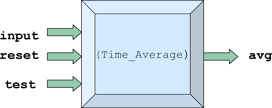File: general_blocks/Statistical/TimeAverage.sim
Description:
The model will take a series of real-valued inputs and find their time average. Each input will be
weighted by the difference in time from when it comes in to the time the next input comes in.

The value of avg is set to zero after each reset input signal is received and also at the beginning
of each simulation.
Ports
Input Ports
• input        Data Type: REAL
• reset         Data Type: TRIGGER
• test           Data Type: TRIGGER
Output Ports
• avg         Data Type: REAL
Parameters
• none

# Weighted_General_MomentsFile: general_blocks/Statistical/Weighted_General_Moments.sim
Description:
The model computes the Weighted N-th moment of a set or real-valued inputs. N is a parameter of the
model.

The model is useful for computing the means and variance from a set of sampled data. The weight input
corresponds to the number of samples from the orginal data that were used to calculate each input to the
model.

Triggering the test input will place the Number of samples currently in the data set onto the num_samples
output port and the computed moment onto the moment_out output port. The sum of the weights of the
samples is placed on the total_weights output.

If the sum of the weights given is zero then the value of the parameter ZeroSampOut will be placed on the
moment_out output instead of trying to compute a moment when no samples are in the set.
Ports
Input Ports
• samp_in      Data Type: REAL
• weight         Data Type: REAL
• reset            Data Type: TRIGGER
• test              Data Type: TRIGGER
Output Ports
• moment_out           Data Type: REAL
• num_samples         Data Type: INTEGER
• total_weights          Data Type: REAL
Parameters
• Moment                            Data Type: INTEGER
• ZeroSampOut = 0.0         Data Type: REAL

# WeightedMeanAndVarianceFile: general_blocks/Statistical/Weighted_MeanAndVariance.sim
Description:
The model computes the mean and variance from a series of real-vaued inputs. The sample variance is
computed as {((n/(n-1)) * [(the 2nd moment of the input values) minus (the square of the sample mean)]}
where n is the number of samples.

If the number of samples is zero, the value of ZeroSampMean parameter is placed on the mean port. If the
number of samples is zero or one, the value of ZeroSampVar parameter is placed on the variance port.

The reset input provides the ability to clear out the entire data set.

Ports
Input Ports
• samp_in     Data Type: REAL
• weight        Data Type: REAL
• reset           Data Type: TRIGGER
• test             Data Type: TRIGGER
Output Ports
• mean                    Data Type: REAL
• variance               Data Type: REAL
• num_samples       Data Type: INTEGER
Parameters
• ZeroSampMean = 0.0      Data Type: REAL
• ZeroSampVar= 0.0          Data Type: REAL

# Global_StatisticsFile: general_blocks/Statistal/Global_Statistics.sim
Description
The model will output the basic statistics computed from
input numbers separated into different (time) batches.
The batches are fixed length in time and are of equal
duration. All inputs which occur before the simulation
clock reaches the value of the StartTime parameter are
discarded. After the start time, the remaining simulation
time is divided into equal length batches and the basic
statistics for all values which come in during a batch
period are placed on the stats output port at the end of
each batch period. The simulation time is divided into
equal time intervals according to two parameters:
NBatches and StartTime.
Ports
Input Ports
• input       Data Type: REAL
Output Ports
• stats     Data Type: Complex Structure "Basic_statistic"
Parameters
• NBatches                      Data Type: INTEGER
• EndTime = 100.0               Data Type: REAL
• NoSampleMean = 0.0            Data Type: REAL
• NoSampleVariance = -1.0       Data Type: REAL